### THE INTEGRATION OF RATIONAL FUNCTIONS, RESULTING IN LOGARITHMIC OR ARCTANGENT FUNCTIONS

The following problems involve the integration of rational functions, resulting in logarithmic or inverse tangent functions. We will assume knowledge of the following well-known differentiation formulas :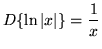,

whererepresents the natural (base e) logarithm of x and,

where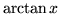represents the inverse function of. These formulas lead immediately to the following indefinite integrals :

1.,
2.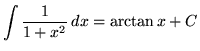.

Because the integral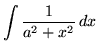,

where a is any positive constant, appears frequently in the following set of problems, we will find a formula for it now using u-substitution so that we don't have to do this simple process each time. Begin by rewriting the function. Then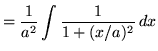.

Now let

u= x/a

so that

du = (1/a) dx ,

or

(a)du = dx .

Now substitute into the original problem, replacing all forms of x, and getting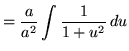.

We now have the following variation of formula 1 :

• 3.Recall also the method of algebraically "completing the square". For example to complete the square with x2+6x we divide 6 by 2, square it getting 9, add and subtract 9, so that

x2+6x = (x2+6x+9) - 9 = (x+3)2 - 9 .

To complete the square with x2+Bx , divide B by 2, square it getting (B/2)2=B2/4, add and subtract B2/4, so that

x2+Bx = (x2+Bx+B2/4) - B2/4 = (x+(B/2))2 - B2/4 .

As you do the following problems, remember these three general rules for integration :,

where n is any constant not equal to -1,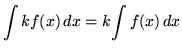,

where k is any constant, and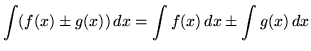.

Most of the following problems are average. A few are challenging. Knowledge of the method of u-substitution will be required on many of the problems. Make precise use of the differential notation dx and du and always be careful when arithmetically and algebraically simplifying expressions. There is a very high emphasis on arithmetic and algebra in this problem set. AVOID MAKING THE FOLLOWING VERY COMMON ALGEBRA MISTAKE :

THIS IS FALSE :.

Convince yourself that this is generally INCORRECT by plugging numbers in for A, B, and C (Try A=4, B=2, and C=2 .).

• PROBLEM 1 : Integrate.

• PROBLEM 2 : Integrate.

• PROBLEM 3 : Integrate.

• PROBLEM 4 : Integrate.

• PROBLEM 5 : Integrate.

• PROBLEM 6 : Integrate.

• PROBLEM 7 : Integrate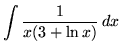.

• PROBLEM 8 : Integrate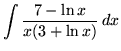.

• PROBLEM 9 : Integrate.

• PROBLEM 10 : Integrate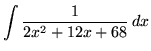.

• PROBLEM 11 : Integrate.

• PROBLEM 12 : Integrate.

• PROBLEM 13 : Integrate.

• PROBLEM 14 : Integrate.

• PROBLEM 15 : Integrate.

• PROBLEM 16 : Integrate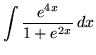.

• PROBLEM 17 : Integrate.

• PROBLEM 18 : Integrate.

• PROBLEM 19 : Integrate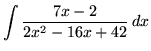.

• PROBLEM 20 : Integrate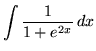.

• PROBLEM 21 : Integrate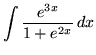.

• PROBLEM 22 : Integrate.